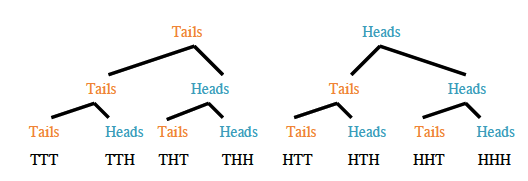### Home > AC > Chapter 14 > Lesson 14.2.1.8 > Problem2-110

2-110.

Toss three coins in the air. Make a list of all possible outcomes and find the probability that:1. All three coins land “heads” up.

$\frac{1}{8}$

2. Two of the coins land “tails” up.

$\frac{3}{8}\text{ or }\frac{1}{2} \text{ assuming TTT counts.}$